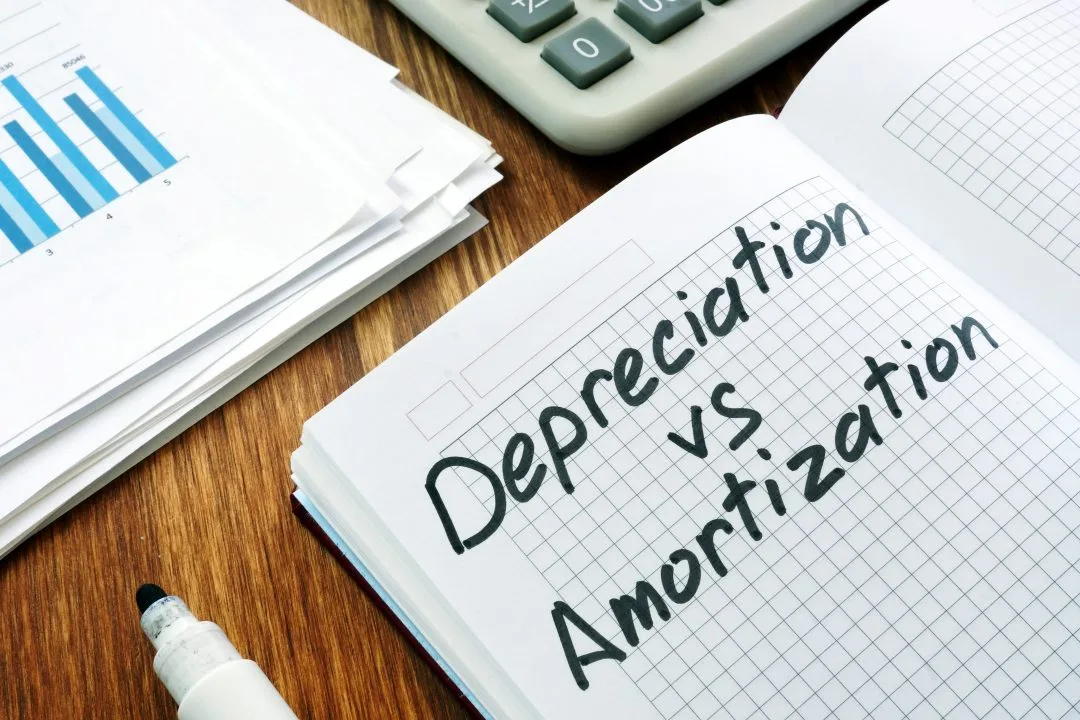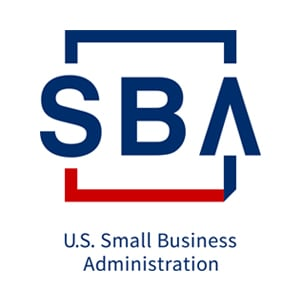# Amortization vs. Depreciation: What’s the Difference?Are you a business owner or investor trying to understand the differences between amortization and depreciation?

These two financial concepts are often used in accounting and finance, but they can be confusing, especially for startups.

Amortization and depreciation are used to spread the cost of a tangible or intangible asset over its useful life. Amortization applies explicitly to intangible assets such as patents and copyrights, while depreciation applies to tangible assets like buildings and equipment.

However, both depreciation and amortization are used to match expenses with revenue to reflect a company’s financial performance more accurately.

Understanding the differences between these two methods can be important for various reasons. For instance, a business owner would want to know the differences between amortization and depreciation because of how it can impact tax liability and the financial statements of their business.

At the same time, an investor may want to know about these differences in order to make informed decisions about the companies they are considering investing in.

This blog post will explain the distinctions between amortization and depreciation, their similarities, and how they are calculated, and will provide examples to help you better understand these concepts.

Let’s delve in!

## What is Amortization?

Amortization refers to the accounting practice of allocating the cost of intangible assets, such as a trademark, franchise agreement, trade name, organizational costs, costs of issuing bonds to raise capital, and patents, over a set period.

This is typically done using the straight-line method, which means that the same amount is recorded as an amortization expense each year over the asset’s useful life. Expensed assets under the amortization method typically don’t have salvage or resale value.

The term “amortization” can also be used in a different, unrelated situation. For example, in the case of a mortgage or a student loan, an amortization schedule is typically used to calculate a series of loan installments that include both principal and interest in each payment.

Despite the differences between the two, the concepts are partially related: because a loan is an intangible good, amortization is the reduction in the carrying value of the balance.

## What is Depreciation?

Depreciation is allocating the cost of a tangible asset, such as a building, furniture, vehicle, or machinery, over its useful life.

Like amortization, depreciation is used to spread out the cost of an asset over time, but it is only applicable to tangible assets.

Contrary to intangible assets, tangible assets may still be valuable after the firm no longer needs them. In order to account for this, depreciation is calculated by deducting the asset’s salvage or resale value from its initial purchase price.

The difference is equally depreciated throughout the asset’s estimated lifespan.

## How to Calculate Depreciation?

To calculate depreciation, you must know the following:

• The cost of the asset, including purchasing the asset, shipping, installation, and training
• The salvage value of an asset at the end of its useful life
• The useful life of the asset

You can find the useful life of specific business assets in Publication 946 How to Depreciate Property.

Once you know the values of the above-bulleted points, your accountant can use several methods, including the straight-line method, the declining balance method, units of production, the double declining balance method, and the sum-of-the-years’-digits method to calculate depreciation for any of your business assets.

## Depreciation Methods

The following are the most common depreciation methods used.

#### Straight-Line Method

The straight-line method is the most frequently used method for calculating depreciation. Under this method, an equal amount of depreciation is recorded each year over the asset’s useful life.

#### Declining Balance Method

The declining balance method calculates depreciation faster than the straight-line method, meaning that a higher percentage of the asset’s value is depreciated in the early years of its useful life.

To calculate this, multiply the asset’s current book value by a fixed depreciation rate that applies over its entire life.

#### Sum-of-the-years’-digits Method

The sum-of-the-years’-digits method is similar to the declining balance method, but the depreciation rate is based on the sum of the digits of the asset’s useful life.

For example, if a tangible asset has a useful life of 15 years (5+4+3+2+1), based on the corresponding digit, a business depreciates a percentage of costs (i.e., 5/15 for Year 1, 4/15 for Year 2, etc.).

#### Double-Declining Balance Method

The double-declining balance method is used by companies when they choose to calculate the depreciation expense of a tangible asset at a faster rate than the straight-line method.

Under this method, the depreciation expense is calculated by taking twice the straight-line depreciation rate and applying it to the current book value of the asset. The asset’s book value is the asset’s original cost minus the accumulated depreciation.

This method is more suitable for assets expected to have a higher usage level and benefits in the early years of their useful lives.

#### Units of Production

This method calculates the depreciation expense of a tangible asset based on its anticipated usage.

For instance, a business might decide to purchase a corporate car and plan to drive it 100,000 miles. It evaluates actual usage each year (12,000 miles traveled in year one) to determine how much depreciation to account for (i.e., 12 % of the depreciable base in year one).

The units of production depreciation method is more accurate than the straight-line method for assets used heavily in the production process, as it more accurately reflects the pattern of an asset’s usage and benefits.

The method used to calculate depreciation can depend on various factors, including the nature of the asset, the length of its useful life, and the company’s accounting policies.

It is essential to choose the method that best reflects an asset’s usage pattern and benefits over its useful life.

## Similarities Between Amortization and Depreciation

Amortization and depreciation are two accounting methods used to spread the cost of a tangible or intangible asset over its useful life.

To aid your understanding of both methods, here are their similarities.

• Both amortization and depreciation are non-cash expenses, meaning they do not involve any cash outflow. Instead, they are recorded as a reduction in the value of an asset on the balance sheet.
• Both amortization and depreciation are recorded as expenses on the income statement.
• Both amortization and depreciation are subject to an impairment or write-off.

## Key Differences Between Amortization and Depreciation

While amortization and depreciation are similar in that they are both accounting methods used to allocate the cost of an asset over the time of its useful life, there are some key differences between them.

Let’s look at some of them below.

#### Applicability of Amortization vs. Depreciation

The difference between amortization and depreciation is clearly seen in their application.

Depreciation calculates the loss of value of a tangible fixed asset over time. Assets owned by the business, such as real estate, tools, structures, buildings, plants, machinery, and cars, can be depreciated.

In contrast, amortization describes the gradual decline in the value of intangible assets such as intellectual property, patents, trademarks, and other comparable assets.

#### Implementation of Amortization vs. Depreciation

The straight-line approach is most frequently used to calculate amortization. In contrast, you can use several methods to calculate depreciation.

For instance, following the depreciation method, you can use the straight-line method (SLM) or accelerated depreciation method, depending on if the asset is the type that depreciates at a steady rate or at a rapid rate in the early years and then depreciates slowly after much usage.

#### Formulae

The formulae for calculating depreciation consider that a tangible item still has some salvage or trash value after its useful life is over.

Salvage value is not included in the amortization formula since an intangible asset lacks this value.

The formulas below illustrate how amortization and depreciation differ from one another.

• Annual Amortization= (Cost of Intangible Asset)/Useful Life.
• Annual Depreciation = (Cost of Tangible Asset – Salvage Value)/Useful Life.

#### Use of Contra Account

Amortization might not use contra assets, whereas depreciation entries always post to Accumulated Depreciation, a contra account to reduce the carrying value of capital assets.

## Examples of Amortization vs. Depreciation

The straight-line method is typically used for calculating amortization. This method records the same amount of amortization each year over the asset’s useful life.

#### The formula for calculating amortization

Amortization expense = (Cost of the asset) / Useful life of the asset

Let’s see a quick example:

If a company buys a patent for \$100,000 with a useful life of 20 years, the annual amortization expense would be calculated as follows:

Amortization expense = (\$100,000) / 20 years = \$5,000 per year

#### The formula for calculating depreciation

For the Depreciation method, the straight-line method can be used as well. Under this method, an equal amount of depreciation is recorded each year over the asset’s useful life.

Depreciation expense = (Cost of an asset – Salvage value) / Useful Life of the asset

Let’s see a quick example:

If an enterprise buys a piece of machinery for \$70,000 with a salvage value of \$10,000 and a useful life of 6 years, the annual depreciation expense would be calculated as follows:

Depreciation expense = (\$70,000 – \$10,000) / 6 years = \$10,000 per year.

## Why Do We Amortize a Loan Rather Than Depreciate a Loan?

Loans are amortized because they are not tangible assets. In contrast to tangible assets, loans do not lose value or wear down like physical assets.

Loans are also amortized because the original asset value has little weight in consideration for a financial statement. Although the notes may have a payment history, a firm only needs to record its current level of debt.

## How Do I Know if I Should Amortize or Depreciate an Asset?

The decision to amortize or depreciate an asset depends on the nature of the asset and its expected useful life.

Typically accounting guidance via GAAP provides accounting instructions on handling different types of assets. Accounting rules stipulate that intangible assets are amortized while physical, tangible assets (except for non-depreciable assets) are to be depreciated.

It’s important to note that the decision to amortize or depreciate an asset is not always straightforward, and it may be necessary to seek the advice of a financial professional.

Understanding the differences between amortization and depreciation is important for businesses and individuals. Here are the key differences:

• Amortization applies to intangible assets, such as franchise agreements, patents, and copyrights, while depreciation applies to tangible assets, such as buildings and equipment.
• Amortization is typically calculated on a straight-line method, while depreciation can be calculated using several methods.
• The tax treatment of amortization and depreciation can differ depending on the type of asset and the applicable tax laws.

Knowing these differences between amortization and depreciation is vital for CEOs and entrepreneurs who might want to sell their company because it can impact the valuation of their company and the taxes they may owe upon the sale.

If you are an entrepreneur looking to sell your company and want to learn more about how amortization and depreciation can impact your deal, schedule a consultation with us or call Sigma Mergers & Acquisitions at (214) 214-9400.

CATEGORIES##### Hi, I’m Scot Cockroft.

When I founded Sigma Mergers and Acquisitions back in 2003, I had sold my business the year prior.

Now, that can sound good, but let me tell you, back in 2003, it was not easy to sell a business. Not that I’m saying in modern day times it’s easy to sell a business, but back then I interviewed broker after broker after broker, and no one was interested in actually seeing the value that my business brought to the table.

SHARE
RELATED POST### What is SDE (Seller’s Discretionary Earnings)?

January 19, 2022### Succession Planning in the Age of Coronavirus

December 22, 2022### CARES Act Impacts SBA 7(a) Loans

December 22, 2022